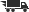###### Error title
Some error text about your books and stuff.
Close

# Mathematics in Action 1

## by Pre-Precalculus Grou Suny A Cuny

• ISBN: 9780201383171
• ISBN10: 0201383179

# Mathematics in Action 1

## by Pre-Precalculus Grou Suny A Cuny

• List Price: \$67.00
• Binding: Paperback
• Publisher: Addison Wesley Publishing Company
• Publish date: 08/01/1998
• ISBN: 9780201383171
• ISBN10: 0201383179
used \$10.35
You save: 85%
Description: (Each chapter ends with a What Have I Learned? & How Can I Practice? section.) I. NUMBER SENSE. 1. Introduction to Problem Solving. Problem-Solving Heuristics. Tables. Pie Charts. Percent. BarGraphs. Estimation. Averages. Rates. Exponents. Scientific Notation. Order of Operations. Grouping Symbols. Distributive Property. 2. Problem Solving with Percents. Fractions. Decimals. Percent. Tables of Data. Ratio and Proportion. Relative Frequency. Probability. Rounding. Histrograms. Inequalities. Estimation. Formulas. Common Formulas. Measurement. Rates. Pie Charts. Inequalities. Numeracy Skills-Check 1. 3. Problem Solving with Fractions. Fractions. Decimals. Percent. Rounding. Averages. Tables of Data. Operations with Fractions. Measurement. Formulas. Estimation. Heuristics. 4. Problem Solving with Ratio and Proportion. Proportions. Rates. Equivalent Fractions. Unit Conversions. Ratio and Proportion. English and Metric Conversion. Order of Magnitude. Exponents. Percent. Rounding. Heuristics. Numeracy Skills-Check 2. 5. Problem Solving with Geometry. Geometry. Formulas. Measurement. Percent. Exponents. Square Roots. Using Formulas. Estimation. Parallelograms. Proportion. Fractions. Decimals. Rates. Unit Conversions. Rounding. Circles. Spheres. Cylinders. Cones. Ratio. Formulas. Percent. Bar Graphs. Averages. Tables of Data. 6. Problem Solving with Signed Numbers. II. VARIABLES: DEVELOPING A SYMBOL SENSE. Signed Numbers. Addition. Subtraction. Opposites. Operations with Signed Numbers. Multiplication. Division. Exponents. Numeracy Skills-Check 3. Part 1 Gateway Review. 1. Interpreting and Constructing Tables and Graphs. Variables. Relationships Defined by Tables. Converting Tables to Graphs. Measurement. Plotting Data Points. Cartesian Coordinates. Labeling Points. Converting Graphs to Tables. 2. Mathematical Modeling Involving a Single Operation. Relationships Defined by Tables and Graphs in Verbal and Symbolic Form. Introduction to Solving Linear Equations. Commutative Property. Evaluating and Solving Equations of the Form y = ax Verbally and Algebraically. Evaluating and Solving Equations of the Form y = x + b. Algebraic Skills Check. 3. Algebraic Expressions Involving Multiple Operations. Algebraic Rules with Two Operations. Generalizing Symbolically. Distributive Property. Order of Operations. Signed Numbers. Formulas. 4. Solving Equations Involving Two or More Operations. Conceptual Basis for Algebraic Equations. Evaluating and Solving Linear Equations of the Form y = ax + b. Tables. Symbolic Rule. 5. Exploring the Distributive Property in Solving Equations. Distributive Property. Factoring Using the Greatest Common Factor. Combining Like Terms. Algebraic Expressions. Solving Linear Equations. Solving Linear Equations Using the Distributive Property. Part 2 Gateway Review. III. FUNCTION SENSE. 1. Introduction to Functions. Numeric, Graphical and Symbolic Representation of Functions. Domain. Range. Interpretation of Graphs. Functions-Increasing, Decreasing, Extrema. 2. Exploring Rate of Change. Interpolation of Graphs. Functions-Increasing, Decreasing. Rate of Change. Rate of Change Notation. 3. Examples of Functions. Numerical, Graphical and Symbolic Representation of Functions. Functions Defined by Graphs. Intercepts. Domain. Range. Extrema. Scaling. Linear Functions. 4. Extending the Domain: Interpolation and Extrapolation. Scaling. Domain. Range. Interpolation. Extrapolation. IV. LINEAR FUNCTIONS. 1. Introduction to Linear Functions. Rate of Change. Slope. Linear Functions-Numerical and Graphical Representation. Intercepts. Scaling. Deriving Equations. Slope-Intercept Form. Break-Even Point. Function Notation. 2. Problem Solving Using Linear Functions. Deriving Equations. Solving Equations Graphically and Algebraically. Inequalities. Deriving an Equation in Slope-Intercept Form from Data. 3. Regression Lines and Systems of Equations Involving Linear Functions. Deriving an Equation in Slope-Intercept Form from Graph and Data. Line of Best Fit. Recognizing Linearity Graphically. Solving Systems of Linear Equations Numerically, Graphically, and by Substitution. Inequalities. Part 4 Gateway Review. Appendix A. Glossary. Answers to Skill Check and Gateway Review Problems. Index.
Expand description
 Seller Condition Comments Price
 Seller: Textbook Exchange ★★★★★ ★★★★★ Location: Oklahoma City, OK Condition: Good Price: \$10.35 Comments:
 Seller: Quality School Texts ★★★★★ ★★★★★ Location: Coshocton, OH Condition: GoodNames on inside cover and numbers on bookedge; no other internal[...] Names on inside cover and numbers on bookedge; no other internal marking/highlighting. Unless specifically stated as present, assume no CD, DVD, access code or other support materials is available. Price: \$10.62 Comments: Names on inside cover and numbers on bookedge; no other internal[...] Names on inside cover and numbers on bookedge; no other internal marking/highlighting. Unless specifically stated as present, assume no CD, DVD, access code or other support materials is available.Please Wait

## Notify Me When Available

Enter your email address below,
and we'll contact you when your school adds course materials for
.
Enter your email address below, and we'll contact you when is back in stock (ISBN: ).